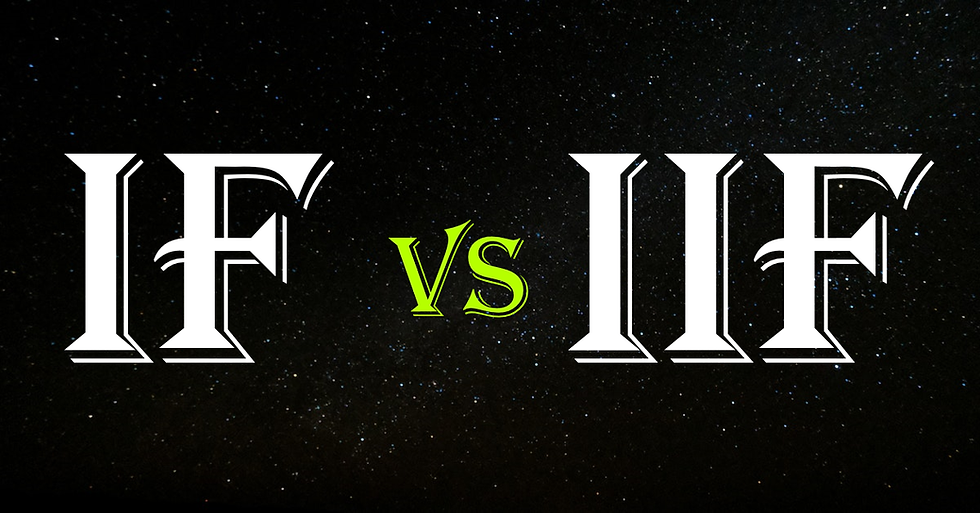top of page
Search

# APPLICATION OF IF AND IIF FUNCTION

Updated: Sep 3, 2020Both IF and IIF functions are logical functions. And like all other logical functions, they allow developers to test whether a certain condition is TRUE or FALSE (boolean logic).

For example, testing whether Sales for different sales agents are below or above target.

While these two functions offer the same solution, there exist a small difference in way of application. Therefore, this article will explore how the two functions differ.

## IF Function

IF function tests an expression, returning one variable if the expression is TRUE and another variable if the expression is FALSE or OTHERWISE.

E.g.

IF(x>0,a,b), the interpretation to this expression is. If x is greater than 0, then return ‘a’ else if x is not greater than zero or x is equal to a null (x<=0 or x=null) then return ‘b’.

Written: If x>0 then ‘a’ else ‘b’ END

## IIF Function

IIF function on the other hand tests an expression, returning one variable if the expression is TRUE and another variable if the expression is FALSE and another variable if the expression is neither TRUE nor FALSE.

Note: This expression returns Null if the third expression is not specified.

Example

IIF(x>0,a,b), the interpretation to this expression is, if x is greater than 0 (x>0) then return ‘a’, else if x is less or equal to zero (X<=0) then return ‘b’, else if x is neither greater than zero (x>0) nor less or equal to zero (x<=0) then ‘Null’.

Written: IIF(x>0,’a’,’b’)

A more complete (IIF) function would be written

IIF(x>0,a,b,c), interpreted as follows; if x is greater than 0 (x>0) then return ‘a’, else if x is less or equal to zero (x<=0) then ‘b’, else if x is neither greater than zero (x>0) nor less or equal to zero (x<=0) then return ‘c’.

Written: IIF(x>0,’a’,’b’,’c’)

## Summary of the functions#Learningtips